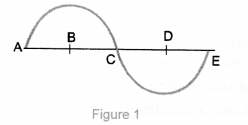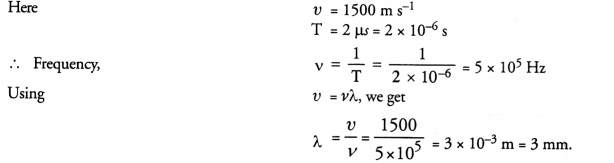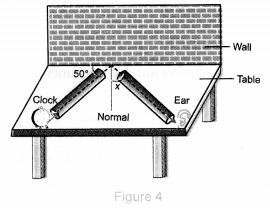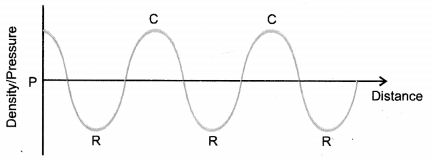## NCERT Exemplar Solutions for Class 9 Science Chapter 12 Sound

These Solutions are part of NCERT Exemplar Solutions for Class 9 Science . Here we have given NCERT Exemplar Solutions for Class 9 Science Chapter 12 Sound

Question 1.
Note is a sound
(a) of mixture of several frequencies
(b) of mixture of two frequencies only
(c) of a single frequency
(d) always unpleasant to listen
(a).

More Resources

Question 2.
A key of a mechanical piano struck gently and then struck again but much harder this time. In the second case
(a) sound will be louder but pitch will not be different
(b) sound will be louder and pitch
(c) sound will be louder but pitch will be lower
(d) both loudness and pitch will remain unaffected
(a). Loudness depends on the amplitude of vibration.

Question 3.
In SONAR, we use
(a) ultrasonic waves
(b) infrasonic waves
(d) audible sound waves
(a).

Question 4.
Sound travels in air if
(a) particles of medium travel from one place to another
(b) there is no moisture in the atmosphere
(c) disturbance moves
(d) both particles as well as disturbance travel from one place to another.
(c).

Question 5.
When we change feeble sound to loud sound we increase its
(a) frequency
(b) amplitude
(c) velocity
(d) wavelength
(b). Loudness depends on the amplitude of vibration.

Question 6.
In the curve (Fig. 1) half the wavelength is(a) A B
(b) B D
(c) D E
(d) A E
(b).

Question 7.
Earthquake produces which kind of sound before the main shock wave begins
(a) ultrasound
(b) infrasound
(c) audible sound
(d) none of the above
(b).

Question 8.
Infrasound can be heard by
(a) dog
(b) bat
(c) rhinoceros
(d) human beings.
(c).

Question 9.
Before playing the orchestra in a musical concert, a sitarist tries to adjust the tension and pluck the string suitably. By doing so, he is adjusting
(a) intensity of sound only
(b) amplitude of sound only
(c) frequency of the sitar string with the frequency of other musical instruments
(d) loudness of sound.
(c).

Question 10.
The given graph (Fig. 2) shows the displacement versus time relation for a disturbance travelling with velocity of 1500 the wavelength of the disturbance.Question 11.
Which of the above two graphs (a) and (b) (Fig. 3) representing the human voice is likely to be the male voice ? Give reason for your answer.Frequency of male voice is lesser than that of female voice. So, graph (a) represents the human voice.

Question 12.
A girl is sitting in the middle of a park of dimension 12 m x 12 m. On the left side of it, there is a building adjoining the park and on the right side of the park, there is a road adjoining by park. A sound is produced on the road by a cracker. Is it possible for the girl to hear the echo of this sound ? Explain your answer.
To hear echo, the distance between the source of sound and the obstacle (i.e., building) should be more than 17 m. In this case, distance between source of sound (i.e., cracker) and the obstacle (i.e., building) is 12 m i.e., less than 17 m. Hence, girl cannot hear the echo of the sound of the cracker.

Question 13.
Why do we hear the sound produced by the humming bees while the sound of vibrations of pendulum is not heard ?
This is because the frequency of sound produced by humming bees lies in audible range (20 Hz to 20,000 Hz) and frequency of the sound of vibrations of pendulum lies in infrasonic region. ( 1 Hz to 19 Hz)

Question 14.
If any explosion takes place at the bottom of a lake, what type of shock waves in water will take place ?
Longitudinal wave as transverse wave cannot travel in water.

Question 15.
Sound produced by a thunderstorm is heard 10 s after the lightning is seen. Calculate the approximate distance of the thunder cloud. (Given speed of sound = 340 m s-1).
Distance of thunder cloud = speed of sound x time = 340 x 10 = 3400 m = 3.4 km.

Question 16.
For hearing the loudest ticking sound heard by the ear, find the angle x in the Fig. 4.Question 17.
Why is the ceiling and wall behind the stage of good conference halls or concert halls made curved ?
So that sound after reflection reach to every audience.

Question 18.
Represent graphically by two separate diagrams in each case :
(i) Two sound waves having the same amplitude but different frequencies ?
(ii) Two sound waves having the same frequency but different amplitudes.
(iii) Two sound waves having the different amplitude but different wavelengths.Question 19.
Establish the relationship between speed of sound, its wavelength and frequency. If velocity of sound in air is 340 m s-1, calculate
(i) wavelength when frequency is 256 Hz.
(ii) frequency when wavelength is 0.85 m. (CBSE 2012)